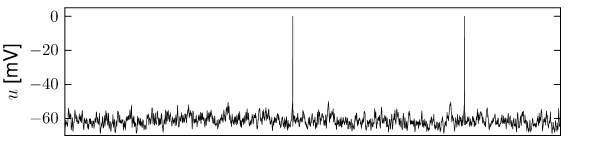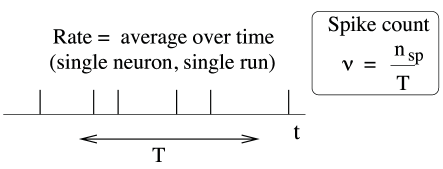# 7.2 Mean Firing Rate

In the next few sections, we introduce some important concepts commonly used for the statistical description of neuronal spike trains. Central notions will be the interspike interval distribution, (Section 7.3), the noise spectrum (Section 7.4), but most importantly the concept of ‘firing rate’ which we discuss first.Fig. 7.5: Variability in a deterministic model network of 8000 excitatory and 2000 inhibitory neurons, both modeled as leaky integrate-and-fire neurons. A. Voltage trace as a function of time for a single model neuron. Spikes (vertical lines) are generated whenever the membrane potential (solid line) hits the firing threshold. B. Spike raster of 100 neurons in the network. Spike times (dots) of a single neuron appear along a horizontal line. C. Population activity A⁢(t)A(t) as a function of time, measured by averaging across the spikes of the subpopulation of 100 neurons shown in B. From time t=1t=1 s to t=3t=3 s, all neurons in this population receive a nonzero input. D. Input to the subpopulation of 100 neurons. Simulation results courtesy of F. Zenke and T. Vogels (539).

A quick glance at the experimental literature reveals that there is no unique and well-defined concept of ‘mean firing rate’. In fact, there are at least three different notions of rate which are often confused and used simultaneously. The three definitions refer to three different averaging procedures: either an average over time, or an average over several repetitions of the experiment, or an average over a population of neurons. The following three subsections will revisit in detail these three concepts.

# 7.2.1 Rate as a Spike Count and Fano Factor

The first and most commonly used definition of a firing rate refers to a temporal average. An experimentalist observes in trial $k$ the spikes of a given neuron (see Fig. 7.6). The firing rate in trial $k$ is the spike count $n_{k}^{\rm sp}$ in an interval of duration $T$ divided by $T$.

 $\nu_{k}={n_{k}^{\rm sp}\over T}\,.$ (7.1)

The length $T$ of the time window is set by the experimenter and depends on the type of neuron and the stimulus. In practice, to get sensible averages, several spikes should occur within the time window. Typical values are $T=100$ ms or $T=500$ ms, but the duration may also be longer or shorter.

This definition of rate has been successfully used in many preparations, particularly in experiments on sensory or motor systems. A classical example is the stretch receptor in a muscle spindle (9). The number of spikes emitted by the receptor neuron increases with the force applied to the muscle.Fig. 7.6: The spike count measure: Definition of the mean firing rate by temporal average.

If the same experiment is repeated several times, the measured spike count varies between one trial and the next. Let us denote the spike count in trial $k$ by the variable $n^{\rm sp}_{k}$, its mean by $\langle n^{\rm sp}\rangle$ and deviations from the mean as $\Delta n^{\rm sp}_{k}=n^{\rm sp}_{k}-\langle n^{\rm sp}\rangle$. Variability of the spike count measure is characterized by the Fano Factor, defined as the variance of the spike count $\langle(\Delta n^{\rm sp})^{2}\rangle$ divided by its mean

 $F={\langle(\Delta n^{\rm sp})^{2}\rangle\over\langle n^{\rm sp}\rangle}\,.$ (7.2)

In experiments, the mean and variance are estimated by averaging over $K$ trials $\langle n^{\rm sp}\rangle=(1/K)\sum_{k=1}^{K}\,n^{\rm sp}_{k}$ and $\langle(\Delta n^{\rm sp})^{2}\rangle=(1/K)\sum_{k=1}^{K}\,(\Delta n^{\rm sp}_% {k})^{2}$.

If we find on average $\langle n^{\rm sp}\rangle$ spikes in a long temporal window of duration $T$, the mean interval between two subsequent spikes is $T/\langle n^{\rm sp}\rangle$. Indeed, using the notion of interspike-interval distribution to be introduced below (Section 7.3), we can make the following statement: the firing rate defined here as spike count divided by the measurement time $T$ is identical to the inverse of the mean interspike interval. We will come back to interspike intervals in Section 7.3.

It is tempting, but misleading, to consider the inverse interspike interval as a ’momentary firing rate’: if a first spike occurs at time $t^{(k)}$ and the next one at time $t^{(k+1)}$, we could artificially assign a variable $\tilde{\nu}(t)=1/(t^{(k+1)}-t^{(k)})$ for all times $t^{(k)}. However, the temporal average of $\tilde{\nu}(t)$ over a much longer time $T$ is not the same as a the mean rate $\nu$ defined here as spike count divided by $T$, simply because $1/\langle x\rangle\neq\langle(1/x)\rangle$. A practical definition of ’instantaneous firing rate’ will be given below in Section 7.2.2.

Example: Homogeneous Poisson Process

If the rate $\nu$ is defined via a spike count over a time window of duration $T$, the exact firing time of a spike does not matter. It is therefore tempting to describe spiking as a Poisson process where spikes occur independently and stochastically with a constant rate $\nu$.

Let us divide the duration $T$ (say 500ms) into a large number of short segments $\Delta t$ (say $\Delta t=0.1$ms). In a homogeneous Poisson process, the probability to find a spike in a short segment of duration $\Delta t$ is

 $P_{F}(t;t+\Delta t)=\nu\,\Delta t\,.$ (7.3)

In other words, spike events are independent of each other and occur with a constant rate (also called stochastic intensity) defined as

 $\nu=\lim_{\Delta t\to 0}{P_{F}(t;t+\Delta t)\over\Delta t}\,.$ (7.4)

The expected number of spikes to occur in the measurement interval $T$ is therefore

 $\langle n^{\rm sp}\rangle=\nu\,T,$ (7.5)

so that the experimental procedure of (i) counting spikes over a time $T$ and (ii) dividing by $T$ gives an empirical estimate of the rate $\nu$ of the Poisson process.

For a Poisson process, the Fano factor is exactly one. Therefore, measuring the Fano factor is a powerful test so as to find out whether neuronal firing is Poisson-like; see the discussion in Rieke et al. (436).

# 7.2.2 Rate as a Spike Density and the Peri-Stimulus-Time Histogram

An experimenter records from a neuron while stimulating with some input sequence. The same stimulation sequence is repeated several times and the neuronal response is reported in a Peri-Stimulus-Time Histogram (PSTH) with bin width $\Delta t$; see Fig. 7.7. The time $t$ is measured with respect to the start of the stimulation sequence and $\Delta t$ defines the time bin for generating the histogram, it is typically on the order of milliseconds.

The number of occurrences of spikes $n_{K}(t;t+\Delta t)$ summed over all repetitions of the experiment divided by the number $K$ of repetitions is a measure of the typical activity of the neuron between time $t$ and $t+\Delta t$. A further division by the interval length $\Delta t$ yields the spike density

 $\rho(t)={1\over\Delta t}{n_{K}(t;t+\Delta t)\over K}\,.$ (7.6)

Sometimes the result is smoothed to get a continuous (time-dependent) rate variable, usually reported in units of Hz. As an experimental procedure, the PSTH measure is a useful method to evaluate neuronal activity, in particular in the case of time-dependent stimuli; see Fig. 7.4. We call it the time-dependent firing rate.Fig. 7.7: The Peri-Stimulus-Time Histogram (PSTH) and the time-dependent firing rate as an average over several runs of the experiment.

In order to see the relation of Eq. 7.6 to a time-dependent firing rate, we recall that spikes are formal events characterized by their firing time $t^{(f)}$ where $f$ counts the spikes. In Chapter 1 we have defined (Eq. (1.14)) the spike train as a sum of $\delta$-functions:

 $S(t)=\sum_{f}\delta(t-t^{(f)})\,.$ (7.7)

If each stimulation can be considered as an independent sample from the identical stochastic process, we can define an instantaneous firing rate as an expectation over trials

 $\nu(t)=\langle S(t)\rangle.$ (7.8)

An expectation value over $\delta$-functions may look weird to the reader not used to seeing such mathematical objects. Let us therefore consider the experimental procedure to estimate the expectation value. First, in each trial $k$, we count the number of spikes that occur in a short time interval $\Delta t$ by integrating the spike train over time, $n^{\rm sp}_{k}(t)=\int_{t}^{t+\Delta t}S_{k}(t^{\prime}){\text{d}}t^{\prime}$ where the lower index $k$ denotes the trial number. Note that integration removes the $\delta$-function. Obviously, if the time bin $\Delta t$ is small enough we will find at most one spike so that $n^{\rm sp}_{k}$ is either zero or one. Second, we average over the $K$ trials and divide by $\Delta t$ in order to have the empirical estimate

 $\nu(t)={1\over K\,\Delta t}\,\sum_{k=1}^{K}n^{\rm sp}_{k}(t)\,.$ (7.9)

The PSTH, defined as spike count per time bin averaged over several trials and divided by the bin length (the right-hand side of Eq. (7.9)), provides therefore an empirical estimate of the instantaneous firing rate (the left-hand side).Fig. 7.8: A. A postsynaptic neuron receives spike input from the population mm with activity AmA_{m}. B. The population activity is defined as the fraction of neurons that are active in a short interval [t,t+Δ⁢t][t,t+\Delta t] divided by Δ⁢t\Delta t.

Example: Inhomogeneous Poisson process

An inhomogeneous Poisson process can be used to describe the spike density measured in a PSTH. In an inhomogeneous Poisson process, spike events are independent of each other and occur with an instantaneous firing rate

 $\nu(t)=\lim_{\Delta t\to 0}{P_{F}(t;t+\Delta t)\over\Delta t}\,.$ (7.10)

Therefore, the probability to find a spike in a short segment of duration $\Delta t$, say, a time bin of 1ms, is $P_{F}(t;t+\Delta t)=\nu(t)\,\Delta t$  . More generally, the expected number of spikes in an interval of finite duration $T$ is $\langle n^{\rm sp}\rangle=\int_{0}^{T}\nu(t)\,dt$ and the Fano factor is one, as was the case for the homogeneous Poisson process.

Once we have measured a PSTH, we can always find an inhomogeneous Poisson process which reproduces the PSTH. However, this does not imply that neuronal firing is Poisson-like. A Poisson process has, for example, the tendency to generate spikes with very short interspike intervals, which cannot occur for real neurons because of refractoriness.

# 7.2.3 Rate as a Population Activity (Average over Several Neurons)

The number of neurons in the brain is huge. Often many neurons have similar properties and respond to the same stimuli. For example, neurons in the primary visual cortex of cats and monkeys are arranged in columns of cells with similar properties (230). Let us idealize the situation and consider a population of neurons with identical properties. In particular, all neurons in the population should have the same pattern of input and output connections. The spikes of the neurons in a population $m$ are sent off to another population $n$. In our idealized picture, each neuron in population $n$ receives input from all neurons in population $m$. The relevant quantity, from the point of view of the receiving neuron, is the proportion of active neurons in the presynaptic population $m$; see Fig. 7.8A. Formally, we define the population activity

 $A(t)={1\over\Delta t}{n_{\rm act}(t;t+\Delta t)\over N}={1\over\Delta t}{\int_% {t}^{t+\Delta t}\sum_{j}\sum_{f}\,\delta(t-t_{j}^{(f)})\,{\text{d}}t\over N}$ (7.11)

where $N$ is the size of the population, $n_{\rm act}(t;t+\Delta t)$ is the number of spikes (summed over all neurons in the population) that occur between $t$ and $t+\Delta t$ where $\Delta t$ is a small time interval; see Fig. 7.8. Eq. (7.11) defines a variable with units inverse time – in other words, a rate.

As we can see from Fig. 7.5C, the population activity may vary rapidly and can reflect changes in the stimulus conditions nearly instantaneously. Before we discuss the problem of neural coding (Section 7.6), let us first study further statistical measures of spike train statistics.